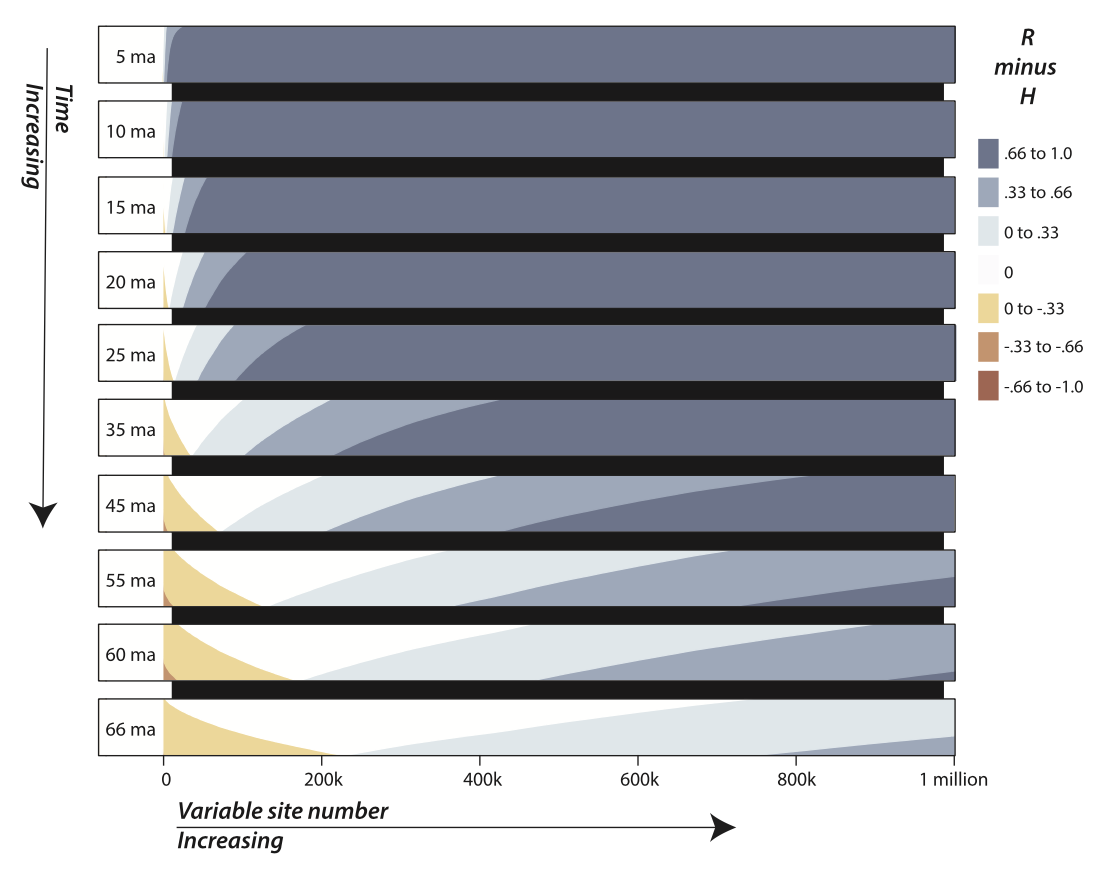Southern Chile, South America

Horizon plots of sequence data

Visualizing the predicted benefits of adding more data

Sequence Data Horizons

We recently finished a study looking at predicted levels of phylogenetic information content in RADseq data. One of the figures in the paper involved using horizon plots to compare the probability of correctly resolving a small internode at different time depths:Horizon plots are a convenient way to display information content as a function of increasing sequence length while being able to simultaneously compare different time periods. These are normally used in time series data and an excellent tutorial on their general use can be found here.

Here is a walkthrough of how to do this in PhyInformR

This tutorial is for users already familiar with PhyInformR. If you haven’t gone through the tutorial, give it a whirl! To start just read in this function, this is going to do the hard work. We will also load tidyverse for a downstream data cleanup.

library(tidyverse)

horizon.prep<-function(rates,pre_name,filename,increment, time, internode,state_space){
nonzero <-rates[which(rates>0)]
max<-length(nonzero)
start<-nonzero[1:increment]
range<-seq(0,max,increment)
outsa<-matrix(ncol=1, nrow=length(range))
outsb<-matrix(ncol=1, nrow=length(range))
outsc<-matrix(ncol=1, nrow=length(range))
for(i in 2:length(range)){
stop<-range[i]
start<-nonzero[1:stop]
start2<-as.matrix(start)
all<-Approximator(time,internode,start2,4)
signal.to.noise<- all-all
begin<-stop-increment

outsc[i,]<-signal.to.noise
outsa[i,]<-begin
outsb[i,]<-stop
}
nn<-rep(pre_name,length(range))
final<-cbind(nn,outsa,outsb,outsc)
write.csv(final, file=filename)
return(final)
}

Let’s demystify this a bit.
rates: You need to feed this your site rates just like any other PhyInformR function.
pre_name: This will keep your times straight in the csv. go with pre_name=”T_yourtime” where your time is the depth of the tree (e.g., 5, 10, 40, 500, etc)
filename: filename=”yourfilename”
increment: how many more sites you want to sample per iteration (e.g., every 50, 100, 500 bp)
time: depth of the tree (T)
internode: internode length (t)
state_space: how many different types of characters you have, (e.g., 4 for nucleotides).

Note that here we are using the files from Prum et al. (2015), which are available on Zenodo (DOI 10.5281) though you could also substitute site rates from your own markers here.

For the purpose of the tutorial I have only read a portion of the site rates (ratesA in the zenodo directory), in this case 27,222 of the sites.

Now we can use these rates and make some plots for resolving a small internode 20,30,40 or 50 million years ago. For the purpose of tutorial I will only test increments of 1000 sites. Also note that the Prum et al guide tree was based on a tree with a root height of 1, so we are going to pick some relative times for the sake of tutorial. With your own trees the T and t values should reflect the scale your guide tree was based on.

library(PhyInformR)
output<-NULL
targetTimes<-c(20,30,40,50,60,70,80)
for (i in 1:length(targetTimes)){
filename<-paste(targetTimes[i],"million", sep="_")
prename<-paste0("T",targetTimes[i])
time<-targetTimes[i]/100
temp<-horizon.prep(ratesA,pre_name= prename,filename=filename,500, time, .03,4)
temp<-na.omit(temp)
output<-cbind(output,as.numeric(temp[,4]))
}

We need a few libriaries to plot these so load these really fast

require(lattice)
require(reshape2)
require(latticeExtra)
require(quantmod)

Almost there! Above you should have csv outputs of each step so you don’t have to repeat the calculations each time and just read those in if you need to plot again. For plotting we first add some column names.

colnames(output)<-c("twenty","thirty","forty","fifty","sixty","seventy","eighty")

Now plot and add some separation between graphs with small white band

horizonplot(ts(output), horizonscale=.3, origin=0,colorkey = TRUE, layout=c(1,7))+

layer(panel.xblocks(height=0.005,col="white",...))

You should have something that looks like this:Note that in order for this to makes sense, you should keep either T or t constant. For example, you could flip this logic and ask for a tree of depth X (e.g., 30 million years) what is the predicted benefit of adding more data for resolving an internode of length Y, Z, etc.

That’s it! From here you can keep modifying the above, breaking out different subsets of data such as sites, or begin to use your favorite graphic editing software to customize as you like. We are working on a new release of PhyInformR that will have a more elegant version of the above as well as a lot of new theory for data exploration, so check back for updates.

Citations

If you use this tutorial and code in a project, please cite:

Near, T.J., MacGuigan, D., Parker, C.E., Jones, C., Struthers, C., Dornburg, A. 2018. Phylogenetic analysis of Antarctic notothenioids illuminates the utility of RADseq for resolving Cenozoic adaptive radiations. Molecular Phylogenetics and Evolution 129: 268—279

and

Dornburg, A., Tamagnon, J., Townsend, J.P. 2016. PhyInformR: Phylogenetic experimental design and phylogenomic data exploration. BMC Evolutionary Biology 16 (1): 262